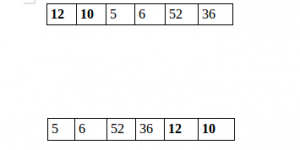Open in App
Not now

# Python Program to Split the array and add the first part to the end

• Difficulty Level : Medium
• Last Updated : 21 Mar, 2023

There is a given an array and split it from a specified position, and move the first part of array add to the end.Examples:

```Input : arr[] = {12, 10, 5, 6, 52, 36}
k = 2
Output : arr[] = {5, 6, 52, 36, 12, 10}
Explanation : Split from index 2 and first
part {12, 10} add to the end .

Input : arr[] = {3, 1, 2}
k = 1
Output : arr[] = {1, 2, 3}
Explanation : Split from index 1 and first

Method 1:

## Python3

 `# Python program to split array and move first` `# part to end.`   `def` `splitArr(arr, n, k): ` `    ``for` `i ``in` `range``(``0``, k): ` `        ``x ``=` `arr[``0``]` `        ``for` `j ``in` `range``(``0``, n``-``1``):` `            ``arr[j] ``=` `arr[j ``+` `1``]` `        `  `        ``arr[n``-``1``] ``=` `x` `        `    `# main` `arr ``=` `[``12``, ``10``, ``5``, ``6``, ``52``, ``36``]` `n ``=` `len``(arr)` `position ``=` `2`   `splitArr(arr, n, position)`   `for` `i ``in` `range``(``0``, n): ` `    ``print``(arr[i], end ``=` `' '``)`   `# Code Contributed by Mohit Gupta_OMG <(0_o)>   `

Output

`5 6 52 36 12 10 `

Time complexity: O(nk), where n is the length of the array and k is the number of times the first part of the array needs to be moved to the end.
Auxiliary space: O(1), the program only uses a constant amount of additional memory.

Method 2:

## Python3

 `# Python program to split array and move first` `# part to end.`     `def` `splitArr(a, n, k):` `    ``b ``=` `a[:k]` `    ``return` `(a[k::]``+``b[::])`     `# main` `arr ``=` `[``12``, ``10``, ``5``, ``6``, ``52``, ``36``]` `n ``=` `len``(arr)` `position ``=` `2` `arr ``=` `splitArr(arr, n, position)` `for` `i ``in` `range``(``0``, n):` `    ``print``(arr[i], end``=``' '``)`

Output

`5 6 52 36 12 10 `

Time Complexity: O(n), where n is the length of the input array ‘arr’.
Auxiliary Space: O(k), where k is the value of ‘position’ input parameter.

Method  3: Using slicing and extend() methods

## Python3

 `# Python program to split array and move first` `# part to end.`   `arr ``=` `[``12``, ``10``, ``5``, ``6``, ``52``, ``36``]` `n ``=` `len``(arr)` `position ``=` `2` `x ``=` `arr[:position]` `y ``=` `arr[position:]` `y.extend(x)` `for` `i ``in` `y:` `    ``print``(i, end``=``" "``)`

Output

`5 6 52 36 12 10 `

Time complexity: O(n)
Auxiliary space: O(n)

Method  4: Using list comprehension and modulo : Another approach is to split an array into two parts and add the first part to the end of the second part. This operation is commonly used in programming and can be useful for a variety of applications, such as rotating the elements of an array or implementing circular buffers.

• Define the function split_and_add(arr, n) that takes an array arr and an integer n as input.
• Compute the length of the input array using the len function and store it in a variable arr_len.
• Use a list comprehension to create a new list result of the same length as the input array, where each element of the new list is computed using the formula (i + n) % arr_len where i is the index of the current element in the input array.
• Return the new list result.

## Python3

 `def` `split_and_add(arr, n):` `    ``return` `[arr[(i ``+` `n) ``%` `len``(arr)] ``for` `i ``in` `range``(``len``(arr))]`   `arr ``=` `[``12``, ``10``, ``5``, ``6``, ``52``, ``36``]` `n ``=` `2`   `result ``=` `split_and_add(arr, n)`   `print``(``*``result)`

Output

`5 6 52 36 12 10`

Time complexity: O(n) : where n is length of the input array. This is because we use a list comprehension to create a new list, and this operation takes O(n) time. The modulo operation % takes constant time, so it does not contribute to the overall time complexity.
Auxiliary space: O(n) : we create a new list of the same length as the input array. This list is used to store the results of the computation, so it takes O(n) space in memory.

Please refer complete article on Split the array and add the first part to the end for more details!

My Personal Notes arrow_drop_up
Related Articles